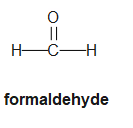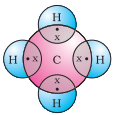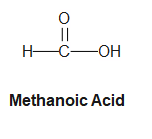# CBSE Important Questions for Carbon Compounds

In this page we have chemical reactions and equations class 10 questions answers. If you need help then you can contact us via e-mail or social media links. Hope you like them and do not forget to like and share the page. a. Multiple Choice Questions
b. One Marks Questions

## Multiple Choice Questions

Question 1.
What is the main component of vinegar?
(a) Ethanol
(b) Methanol
(c) Ethanoic Acid
(d) Water
Question 2.
What type of reaction occurs when ethanoic acid reacts with alcohol?
(a) Esterification
(b) Oxidation
(c) Reduction
(d) Substitution
Question 3.
Which of the following is a property of ethanoic acid?
(a) Sweet Smell
(b) Solid at Room Temperature
(c) Pungent, Vinegar-like Smell
(d) Insoluble in Water
Question 4.

What is the molecular formula of ethanoic acid?
(a) $CH_4$​
(b) $C_2H_6$
​(c) $CH_3COOH$
(d) $C_2H_5OH$
Question 5.
Which of the following is NOT a property of ethanol?
(a) Used as a Disinfectant
(b) Toxic in Large Quantities
(c) Pungent Smell
(d) Active Ingredient in Alcoholic Beverages
Question 6.
What type of bond is present in unsaturated carbon compounds?
(a) Single Bond
(b) Double or Triple Bond
(c) Ionic Bond
(d) None of these
Question 7.
Buckminsterfullerene is an allotropic form of
(a) phosphorus
(b) sulphur
(c) carbon
(d) tin
Question 8.
Carbon forms four covalent bonds by sharing its four valence electrons with four univalent atoms, e.g. hydrogen. After the formation of four bonds, carbon attains the electronic configuration of
(a) helium
(b) neon
(c) argon
(d) krypton
Question 9.
The first member of alkyne homologous series is
(a) ethyne
(b) ethene
(c) propyne
(d) methane

1. (c)
2. (a)
3. (c)
4. (c)
5. (c)
6. (b)
7. (c)
8. (b)
9. (a)

## One Marks Questions

Question 1.
Draw the structure of simplest aldehyde?Question 2.
Name the organic compound which can be produced by fermentation of sugar and is a constituent of beer?

ethanol

Question 3.
Write the name and formula of 2nd member of homologous series having general formula CnH2n-2?

Alkynes and $C_3H_4$(Propyne)

Question 4.
Write the number of covalent bonds in the molecule of butane C4H10?

13

QQuestion 5.
Why soap forms scum with hard water?

The calcium and magnesium ions present in hard water reacts with Soap to form insoluble precipitate which is called scum.So, soap does not form lather in hard water, making its cleansing action less effective in hard water.

Question 6.
Would you able to check if water is hard by using a detergent?

No as they lather easily with both soft and hard water

Question 7.
Why covalent compounds are generally poor conductor of electricity?

Covalent bond is formed by sharing of electrons. There is no ions formation.Hemce poor conductor of electricity

Question 8.
Draw the electron dot and cross structure of the main covalent compound present in compressed natural gas (CNG)?

Methane is the main covalent compound present in CNGQuestion 9.
What type of bond is present in F2?

Covalent bond

Question 10.
State the number of valence electrons and valency of carbon atom?

Carbon has four valence electrons in its outermost shell. Thus, its valency is four.

Question 11.
Write molecular formula of alcohol which can be derived from butane?

$CH_3CH_2CH_2CH_2OH$

Question 12.
Draw the structural formula of methanoic acid?Question 13.
A colourless gas 'X' has a formula C3H6. It decolorizes bromine water? Write the chemical name of 'X' ?
Question 14.
State two characteristics features of carbon which when put together give rise to large number of carbon compounds?
Question 15.
Write the molecular formula of benzene & state the number of double bond in its structure?
Question 16.
Write the name and formula of first member of the carbon compounds having functional group- COOH?
Question 17.
Name the functional group present in –
1. CH3CHO
2. C2H5 COOH
Question 18.
What are oxidizing agents?

Question 19.
Write the molecular formula of the following compound and draw their electron dot structure?
1. Ethane
2. Ethene
3. Ethyne
Question 20.
Explain the mechanism of cleansing action of soap?
Question 21.
List the physical properties of covalent compound and give reason for each?
Question 22.
Why carbon and its compound are used as fuel most applications?
Question 23.
Write balanced chemical equation of the reaction of ethanoic acid with
1. Sodium carbonate
2. Sodium hydrogen carbonate
3. Sodium hydroxide
Question 24.
(i) Give a chemical test to distinguish between alcohol and carboxylic acid.
(ii) Describe soapenification?
Question 25.
Name the process and write the complete reaction involved in the following conversions –
1. Ethanol to ethane
2. Ethanoic acid to ester
3. Ester to ethanol
Question 26.
An organic compound ‘X’ of molecular formula C2H6O on oxidation with alkaline KMnO4 gives a compound ’Y’. On heating compound ‘X’ with compound ‘Y’ in presence of concentrated H2SO4, a sweet smelling compound ‘Z’ in produced. Identify X, Y, Z? Write chemical equation of the above reaction involved?
Question 27.
An organic compound X is an essential constituent of wine and beer ‘X’ is responsible for intoxication caused by thee drinks. Oxidation of ‘X’ yields an organic acid Y which is present in vinegar.
Question 28.
What is a homologous series? Explain with an example?
Question 29.
With the help of an example, explain the process of hydrogenation. Mention the essential conditions for the reaction & state the change I physical properties with the formation of product.